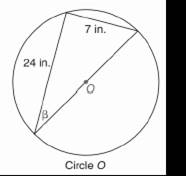Chapter 11.CR, Problem 8CRElementary Geometry For College St...

7th Edition
Alexander + 2 others
ISBN: 9781337614085

Solutions

Chapter
SectionElementary Geometry For College St...

7th Edition
Alexander + 2 others
ISBN: 9781337614085
Textbook Problem

In Review Exercises 5 to 8, state the ratio needed, and use it to find the measure of the indicated angle to the nearest degree.To determine

To find:

The measure of the angle indicated to the nearest degree.

Explanation

Procedure used:

In any right triangle ABC with mA=θ we have the following ratios.

sinθ=OppositeHypotenuse

Calculation:

Given:

The triangle inside the circle is a right triangle since, the diameter subtends right angle at its circumference.

Thus in ΔABC

AB=24 in.,

BC=7 in

Still sussing out bartleby?

Check out a sample textbook solution.

See a sample solution

The Solution to Your Study Problems

Bartleby provides explanations to thousands of textbook problems written by our experts, many with advanced degrees!

Get Started

Find more solutions based on key concepts